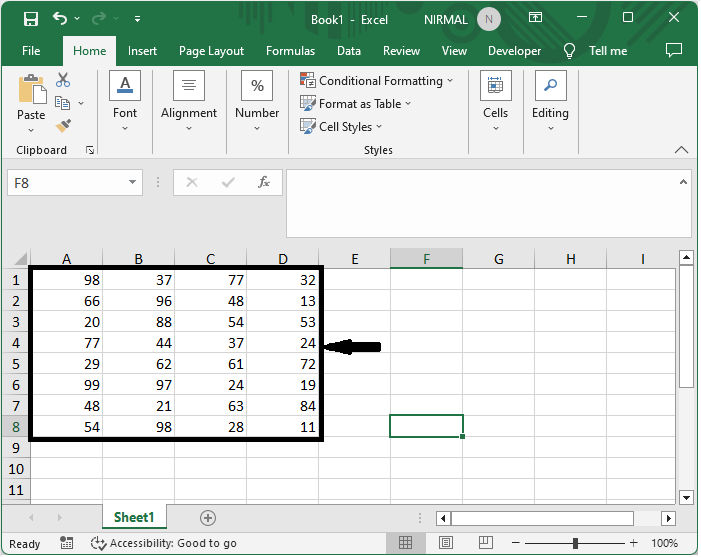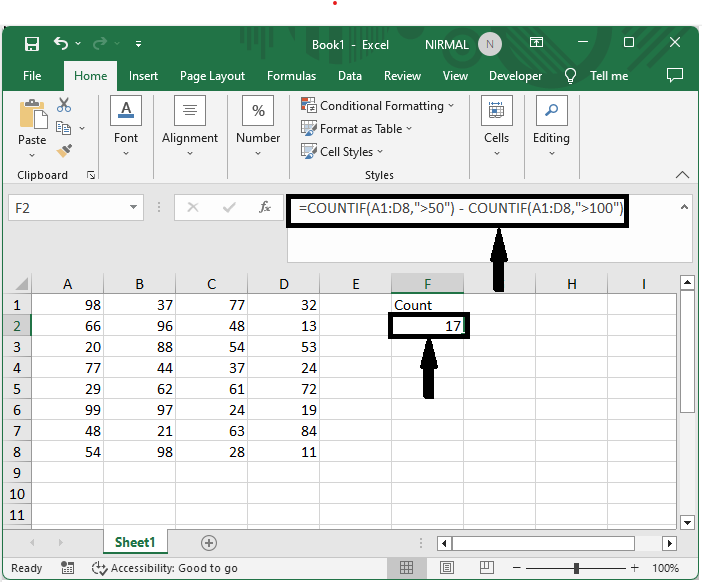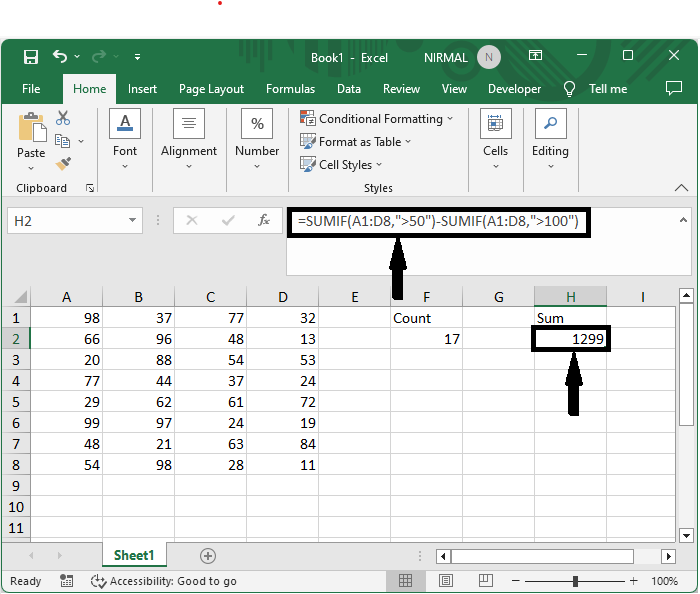# How to Count/Sum the Cells Greater than but Less than a Number?

The situation where you need to count or add the cells that are more than one number but less than another will be the main emphasis of this instruction. When working with huge datasets and need to swiftly extract specific information based on established criteria, this expertise is quite helpful. By the end of this session, you will have a firm grasp on how to use Excel's functions and formulae to effectively count and sum cells that fit the required criteria, streamlining and improving the accuracy of your data analysis activities.

## Count/Sum the Cells Greater than but Less than a Number

Here, we can use the formula directly to complete the task. So let us see a simple process to know how you can count or sum the cells greater than but less than a number in Excel.

### Step 1

Consider an Excel sheet where you have a range of cells with numerical values similar to the below image.First, to count the number of cells, click on an empty cell and enter the formula as =COUNTIF(A1:D8,">50") - COUNTIF(A1:D8,">100") and click enter. In the formula A1:C5, the range of cells is 30, and the bounds are 50.

Empty cell > Formula > Enter.### Step 2

Then, to sum cells, click on an empty cell and enter the formula as =SUMIF(A1:D8,">50")-SUMIF(A1:D8,">100") and click enter. In the formula A1:C5, the range of cells is 30, and the bounds are 50.

Empty cell > Formula > Enter.This is how you can count or sum the cells greater than but less than a number in Excel.

## Conclusion

In this tutorial, we have used a simple example to demonstrate how you can count or sum the cells greater than but less than a number in Excel to highlight a particular set of data.

Updated on: 21-Aug-2023

34 Views